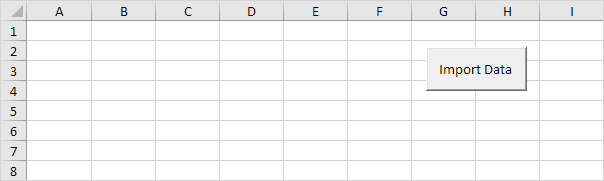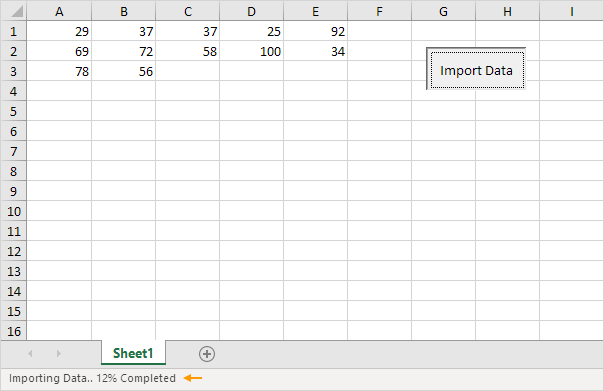# StatusBar

The StatusBar property of the Application object in Excel VBA can be used to indicate the progress of a lengthy macro. This way, you can let the user know that a macro is still running.

Situation:

The macro we are going to create fills Range("A1:E20") with random numbers.Add the following code lines to the command button:

1. First, we declare three variables of type Integer, named i, j and pctCompl.

Dim i As Integer, j As Integer, pctCompl As Integer

For i = 1 To 20
For j = 1 To 5

Next j
Next i

Add the following code lines (at 3, 4 and 5) to the loop.

3. Use the RandBetween function to import a random number between 20 and 100.

Cells(i, j).Value = WorksheetFunction.RandBetween(20, 100)

4. Initialize the variable pctCompl. The second code line writes the value of the variable pctCompl and some descriptive text in the status bar.

pctCompl = (i - 1) * 5 + (j * 1)
Application.StatusBar = "Importing Data.. " & pctCompl & "% Completed"

Example: For i = 3, j = 1, (3 - 1) * 5 + (1 * 1) = 11% has been completed.

5. We use the Wait method of the Application object to simulate a lengthy macro.

Application.Wait Now + TimeValue("00:00:01")

6. To restore the default status bar text, set the StatusBar property to False (outside the loop).

Application.StatusBar = False

Result when you click the command button on the sheet:Note: You can interrupt a macro at any time by pressing Esc or Ctrl + Break. For a more visual approach, see our Progress Indicator program.

Go to Next Chapter: ActiveX Controls